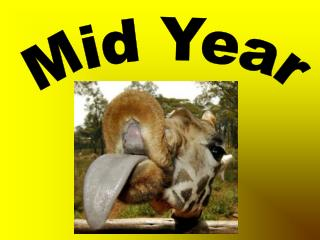DownloadDownload PresentationMid Year

# Mid Year

Download Presentation## Mid Year

- - - - - - - - - - - - - - - - - - - - - - - - - - - E N D - - - - - - - - - - - - - - - - - - - - - - - - - - -
##### Presentation Transcript

1. Mid Year

2. Thirty three thousand two hundred one and one tenth30,000 + 3,000 + 200 +1 +.1

3. 22.9

4. 123 / 1000

5. Write the decimal in order from greatest to least. .123 .12 .32 .3

6. .32 , .3 , .123 , .12

7. Round the numbers to the underlined place values. 54, 653 2.543

8. 55,0002.54

9. \$20.00

10. Estimate the sum by rounding to the nearest whole numbers. 3.2 + 11.76 + 1.5

11. 3 + 12 + 2 = 17

12. Add the decimals. 11.34 +7.33

13. 18.67

14. Subtract the decimals. 423.4 - 5.64

15. 417.76

16. Tony earned \$10 for mowing the lawn, \$15 FOR WASHING THE CAR, AND \$7.00 for cleaning his room. After he got paid he bought a football for \$19.95. How much money will he have left? Write an equation and solve.

17. \$12.05

18. Elliot is having a party and needs to set up tables. if there will be 59 guest and each table seats 8 people, how many tables will be needed?

19. 8 tables

20. Use the model to write a complete division and multiplication equation. n 375 25

21. 25 * n = 375375 / n = 25 n = 15

22. 81 7/9

23. 21 15/21

24. Find the following product: 935 * 8

25. 7,480

26. Find the following product: 321 * 45

27. 14,445

28. 3*3*3*381

29. Find the following product: 36.7 * 9.23

30. 338.741

31. Find the following quotient: 93.6 / 6.4

32. 14.625

33. Find the following Quotient: 8.36 / 4

34. 2.09

35. Find the following Quotient: 135/ 4.5

36. 30

37. Write an estimate and then place the decimal in product. 6.5 * 10.95 = 7 1 1 7 5

38. 7*11 = 77 71.175

39. Write an estimate and then place the decimal in quotient. 8.8 / .22 = 4 0 0 0

40. 9 / .25 =36 40.00

41. Rich makes \$75.00 a day painting . How much will he make if he works for 12 days?

42. \$900.00

43. Tom and his 3 friends won a contest. They want to split the \$864.00 prize money between them. How much will each boy get?